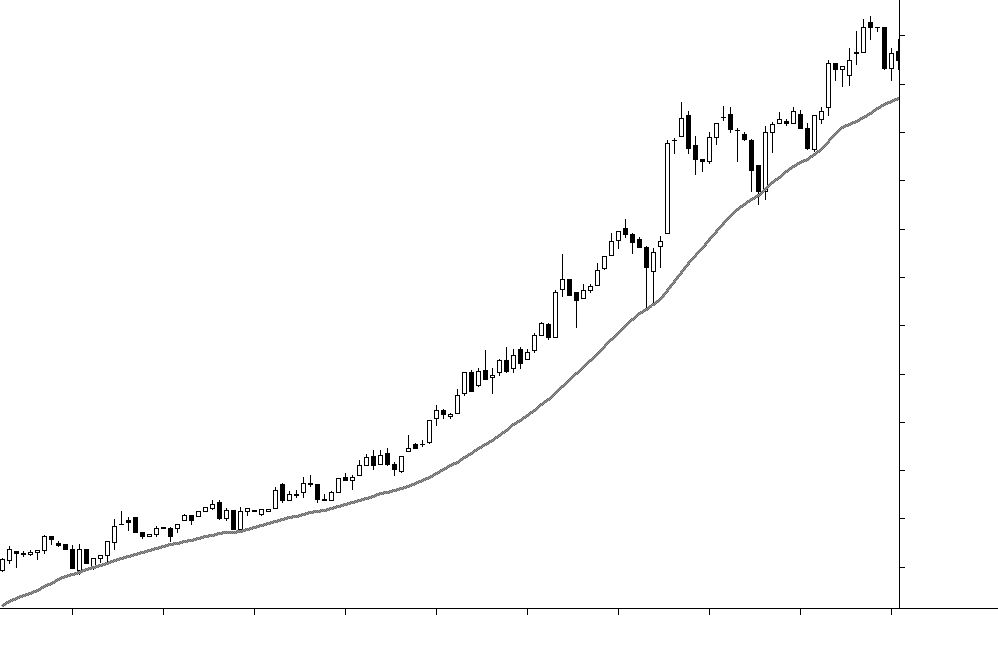Introduction to moving averages

Prior to the advent of personal computers, charting was very much a manual and fairly tedious pursuit and therefore analysis techniques rarely went beyond the application of a few trendlines. However, thanks to the introduction of PCs and software like Stockopedia, we can now generate price charts in a matter of seconds.

Moreover, we can very quickly and easily make fairly complex calculations based on price information in order to design and create a vast range of what we call technical indicators. And the most basic building block of nearly all modern technical indicators is the moving average.

In previous modules I’ve often remarked that chartists need to exercise discretion…an obvious example being the placement of trendlines. But one way to minimize the use of discretion is by defining price trends using moving averages rather than trendlines. Furthermore, because price activity doesn’t always progress in a straight line, it is often more helpful to use moving averages which will ‘bend’ in sympathy with price activity.

A moving average being used as a trendlineIt is important to note that when calculating a moving average it is the convention to use the closing price as, explained in an earlier module, this is considered to be the most significant price component. Hence, all commercially available charting programs will, by default, use the closing price to calculate moving averages.

Simple moving average

A simple moving average (SMA) smoothes out the price activity on a chart by adding together the prices of ‘X’ number of trading periods and then dividing it by ‘X’. As each new trading period occurs, the average is then recalculated and all the averages are then connected using a single unbroken line. So to calculate a simple 5 week moving average the following formula would be applied.

5 Week SMA = Price for week1+week2+week3+week4+week5

The name ‘Simple Moving Average’ is used because it is created using the formula for calculating a ‘Simple Average’. This type of moving average has been in use by chartists since the early 1900s, although you can appreciate how time consuming it was to calculate and draw an SMA by hand.

But as I’ve already mentioned, we have charting software that does it all for us…